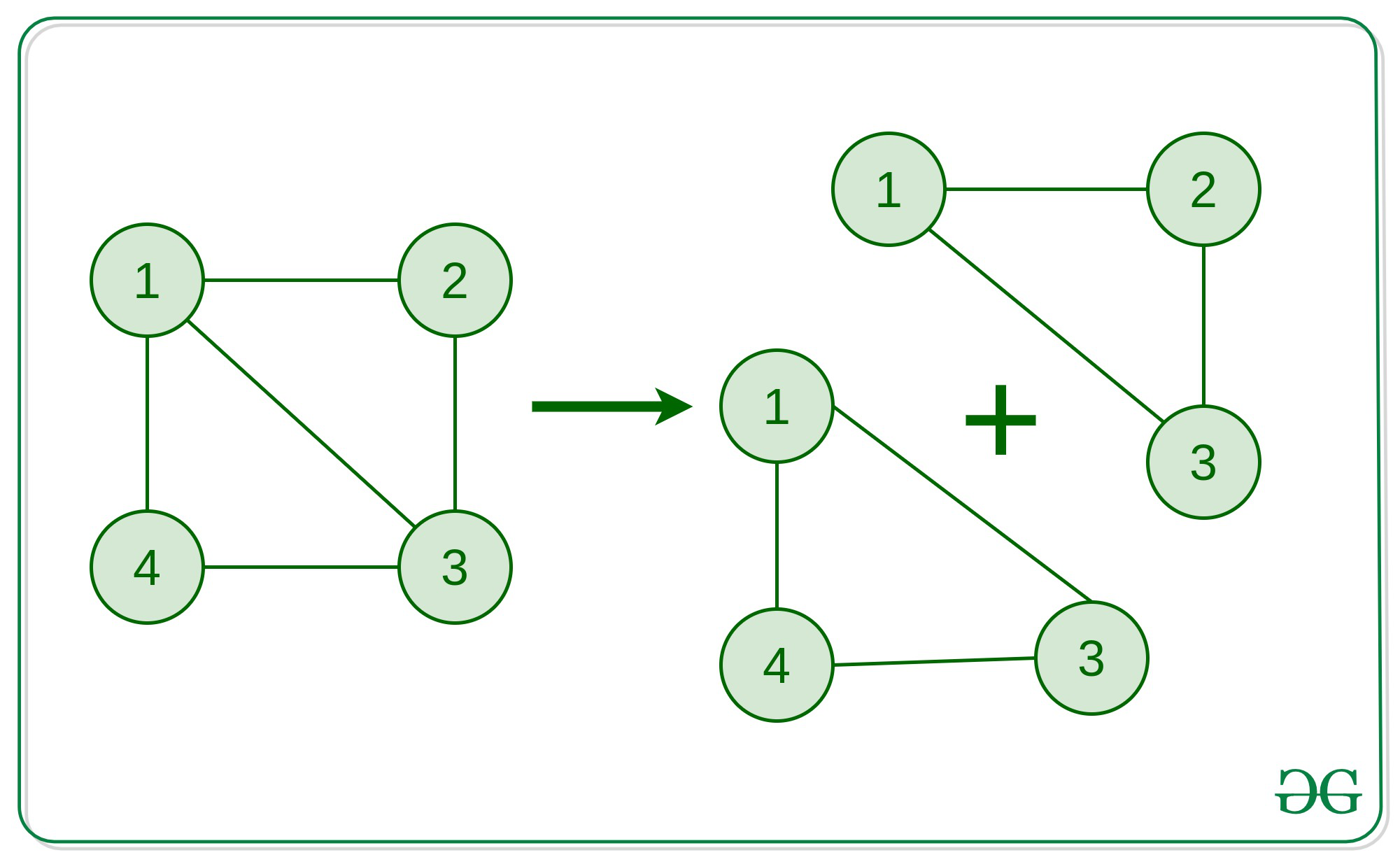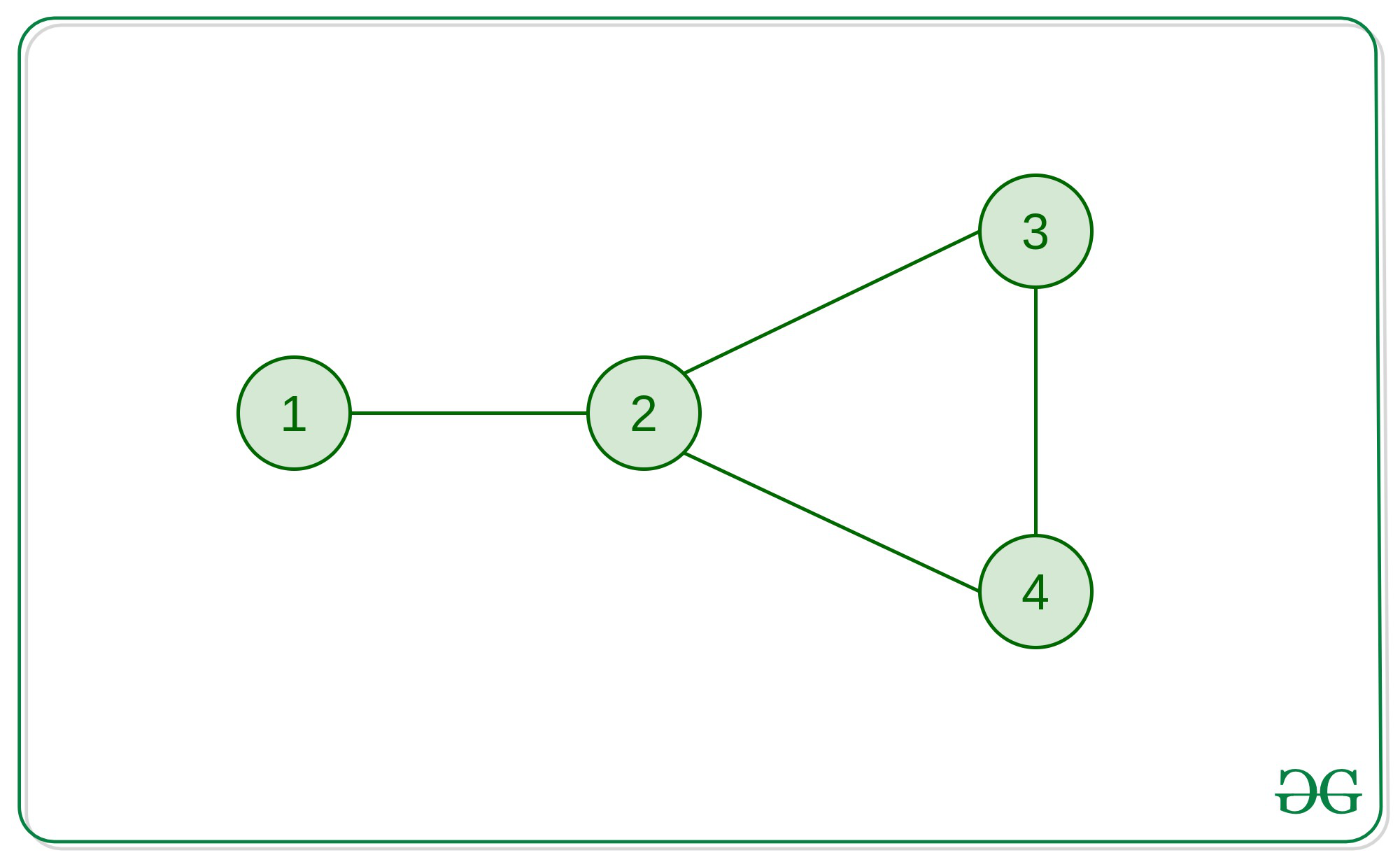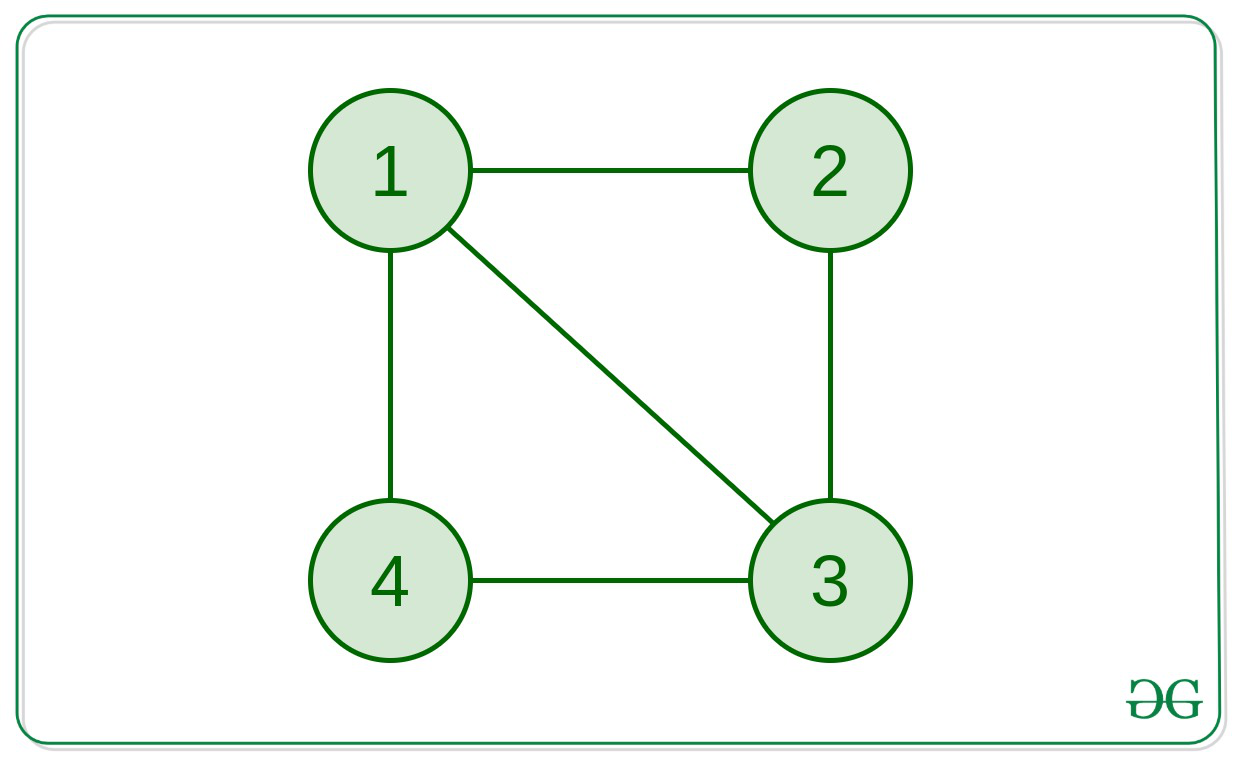# Find any simple cycle in an undirected unweighted Graph

Given an un-directed and unweighted connected graph, find a simple cycle in that graph (if it exists).

Simple Cycle:

A simple cycle is a cycle in a Graph with no repeated vertices (except for the beginning and ending vertex).

Basically, if a cycle can’t be broken down to two or more cycles, then it is a simple cycle.
For better understanding, refer to the following image:The graph in the above picture explains how the cycle 1 -> 2 -> 3 -> 4 -> 1 isn’t a simple cycle
because, it can be broken into 2 simple cycles 1 -> 3 -> 4 -> 1 and 1 -> 2 -> 3 -> 1 .

Examples:

Input: edges[]  = {(1, 2), (2, 3), (2, 4), (3, 4)}Output: 2 => 3 => 4 => 2
Explanation:
This graph has only one cycle of length 3 which is a simple cycle.

Input: edges[] = {(1, 2), (2, 3), (3, 4), (1, 4), (1, 3)}Output: 1 => 3 => 4 => 1

Approach:  The idea is to check that if the graph contains a cycle or not. This can be done by simply using a DFS

Now, if the graph contains a cycle, we can get the end vertices (say a and b) of that cycle from the DFS itself. Now, if we run a BFS from a to b (ignoring the direct edge between a and b), we’ll be able to get the shortest path from a to b, which will give us the path of the shortest cycle containing the points a and b. The path can be easily tracked by using a parent array. This shortest cycle will be a simple cycle.

#### Proof that the shortest cycle will be a simple cycle:

We can prove this using contradiction. Let’s say there exists another simple cycle inside this cycle. This means the inner simple cycle will have a shorter length and, hence it can be said that there’s a shorter path from a to b. But we have found the shortest path from a to b using BFS. Hence, no more shorter path exists and the found path is the shortest. So, no inner cycles can exist inside of the cycle we’ve found.
Hence, this cycle is a simple cycle.

Below is the implementation of the above approach:

## C++

 `// C++ implementation to find the ` `// simple cycle in the given path ` ` `  `#include ` `using` `namespace` `std; ` `#define MAXN 1005 ` ` `  `// Declaration of the Graph ` `vector > adj(MAXN); ` ` `  `// Declaration of visited array ` `vector<``bool``> vis(MAXN); ` `int` `a, b; ` ` `  `// Function to add edges ` `// connecting 'a' and 'b' ` `// to the graph ` `void` `addedge(``int` `a, ``int` `b) ` `{ ` `    ``adj[a].push_back(b); ` `    ``adj[b].push_back(a); ` `} ` ` `  `// Function to detect if the ` `// graph contains a cycle or not ` `bool` `detect_cycle(``int` `node, ``int` `par) ` `{ ` `    ``// Marking the current node visited ` `    ``vis[node] = 1; ` `    ``// Traversing to the childs ` `    ``// of the current node ` `    ``// Simple DFS approach ` `    ``for` `(``auto` `child : adj[node]) { ` `        ``if` `(vis[child] == 0) { ` `            ``if` `(detect_cycle(child, node)) ` `                ``return` `true``; ` `        ``} ` ` `  `        ``// Checking for a back-edge ` `        ``else` `if` `(child != par) { ` `            ``// A cycle is detected ` `            ``// Marking the end-vertices ` `            ``// of the cycle ` `            ``a = child; ` `            ``b = node; ` `            ``return` `true``; ` `        ``} ` `    ``} ` `    ``return` `false``; ` `} ` ` `  `vector<``int``> simple_cycle; ` ` `  `// Function to get the simple cycle from the ` `// end-vertices of the cycle we found from DFS ` `void` `find_simple_cycle(``int` `a, ``int` `b) ` `{ ` `    ``// Parent array to get the path ` `    ``vector<``int``> par(MAXN, -1); ` ` `  `    ``// Queue for BFS ` `    ``queue<``int``> q; ` `    ``q.push(a); ` `    ``bool` `ok = ``true``; ` `    ``while` `(!q.empty()) { ` `        ``int` `node = q.front(); ` `        ``q.pop(); ` `        ``vis[node] = 1; ` `        ``for` `(``auto` `child : adj[node]) { ` `            ``if` `(node == a && child == b) ` `                ``// Ignoring the direct edge ` `                ``// between a and b ` `                ``continue``; ` ` `  `            ``if` `(vis[child] == 0) { ` `                ``// Updating the parent array ` `                ``par[child] = node; ` ` `  `                ``if` `(child == b) { ` `                    ``// If b is reached, ` `                    ``// we've found the ` `                    ``// shortest path from ` `                    ``// a to b already ` `                    ``ok = ``false``; ` `                    ``break``; ` `                ``} ` `                ``q.push(child); ` `                ``vis[child] = 1; ` `            ``} ` `        ``} ` `        ``// If required task is done ` `        ``if` `(ok == ``false``) ` `            ``break``; ` `    ``} ` ` `  `    ``// Cycle starting from a ` `    ``simple_cycle.push_back(a); ` `    ``int` `x = b; ` ` `  `    ``// Until we reach a again ` `    ``while` `(x != a) { ` `        ``simple_cycle.push_back(x); ` `        ``x = par[x]; ` `    ``} ` `} ` ` `  `// Driver Code ` `int` `main() ` `{ ` ` `  `    ``// Creating the graph ` `    ``addedge(1, 2); ` `    ``addedge(2, 3); ` `    ``addedge(3, 4); ` `    ``addedge(4, 1); ` `    ``addedge(1, 3); ` ` `  `    ``if` `(detect_cycle(1, -1) == ``true``) { ` `        ``// If cycle is present ` ` `  `        ``// Resetting the visited array ` `        ``// for simple cycle finding ` `        ``vis = vector<``bool``>(MAXN, ``false``); ` `        ``find_simple_cycle(a, b); ` ` `  `        ``// Printing the simple cycle ` `        ``cout << ``"A simple cycle: "``; ` `        ``for` `(``auto``& node : simple_cycle) { ` `            ``cout << node << ``" => "``; ` `        ``} ` `        ``cout << a; ` `        ``cout << ``"\n"``; ` `    ``} ` `    ``else` `{ ` `        ``cout << ``"The Graph doesn't "` `             ``<< ``"contain a cycle.\n"``; ` `    ``} ` ` `  `    ``return` `0; ` `} `

Output:

```A simple cycle: 1 => 4 => 3 => 1
```My Personal Notes arrow_drop_upCheck out this Author's contributed articles.

If you like GeeksforGeeks and would like to contribute, you can also write an article using contribute.geeksforgeeks.org or mail your article to contribute@geeksforgeeks.org. See your article appearing on the GeeksforGeeks main page and help other Geeks.

Please Improve this article if you find anything incorrect by clicking on the "Improve Article" button below.